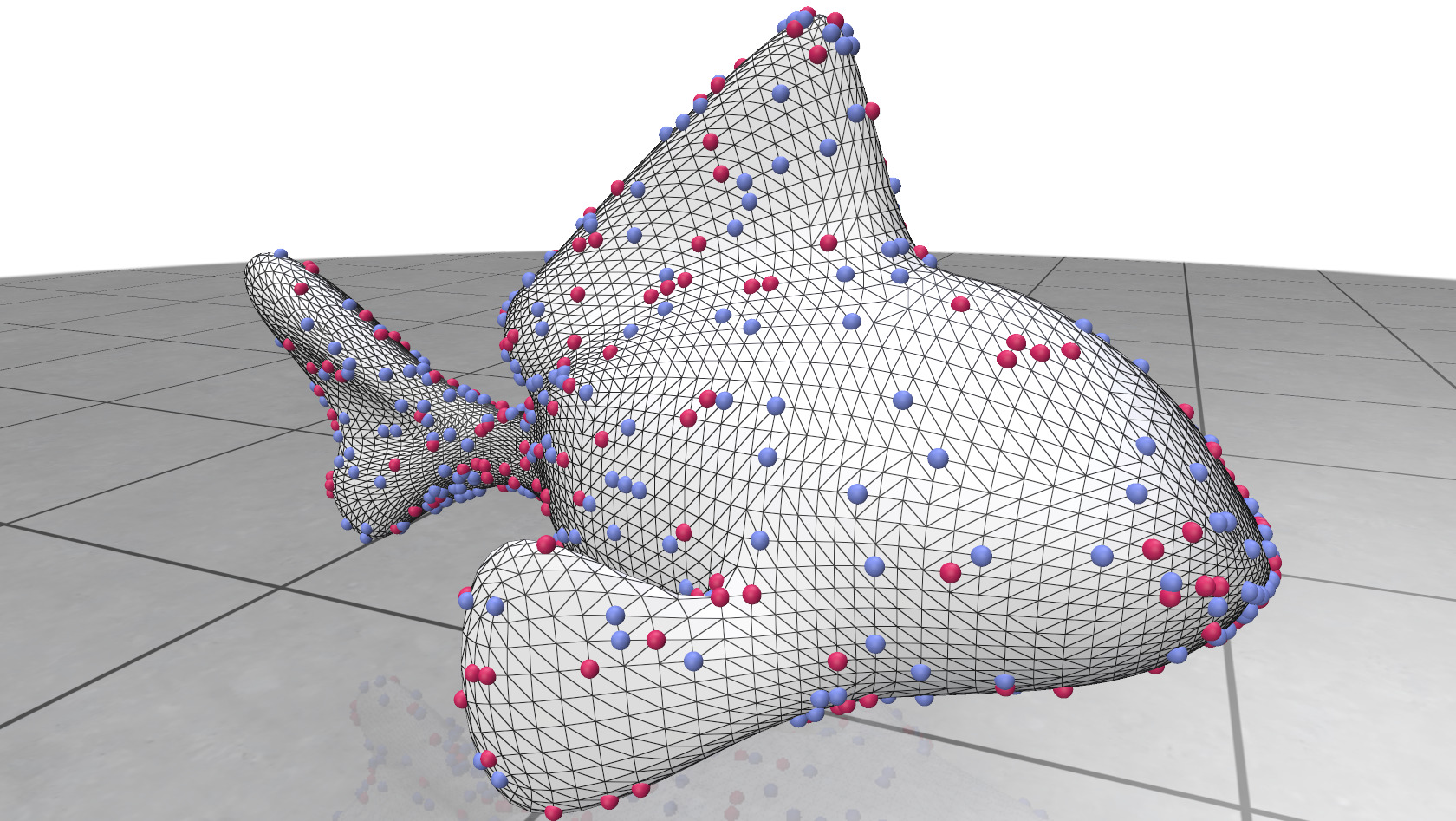# Count Quantities

Count quantities store integers defined at just a few elements of a mesh, rather than at every element. These useful for visualizing special vertices or faces, e.g., singularities of a vector field.Example:

#include "polyscope/polyscope.h"
#include "polyscope/surface_mesh.h"

// Generate a random count quantity
std::vector<std::pair<size_t, int>> vCount;
for (size_t iV = 0; iV < nVertices; iV++) {
if (polyscope::randomUnit() > 0.95) {
vCount.push_back(std::make_pair(iV, 2));
}
}



Unlike almost all other quantity adders in Polyscope, these do not use the data adaptors, and must be explicitly given a std::vector<>.

SurfaceVertexCountQuantity* SurfaceMesh::addVertexCountQuantity(std::string name, const std::vector<std::pair<size_t, int>>& values)

Add a count quantity defined at vertices.

• values is a list of pairs, where the first entry of each pair is a vertex index, and the second is an integer value at that vertex. This list may be any length.
SurfaceFaceCountQuantity* SurfaceMesh::addFaceCountQuantity(std::string name, const std::vector<std::pair<size_t, int>>& values)

Add a count quantity defined at faces.

• values is a list of pairs, where the first entry of each pair is a face index, and the second is an integer value at that vertex. This list may be any length.
SurfaceVertexIsolatedScalarQuantity* SurfaceMesh::addVertexIsolatedScalarQuantity(std::string name, const std::vector<std::pair<size_t, double>>& values)

Add an isolated quantity defined at vertices. This is very similar to the SurfaceVertexCountQuantity, except the data is real-valued rather than integer-valued.

• values is a list of pairs, where the first entry of each pair is a vertex index, and the second is an real value at that vertex. This list may be any length.

### Options

Parameter Meaning Getter Setter Persistent?
enabled is the quantity enabled? bool isEnabled() setEnabled(bool newVal) yes
color map the color map to use std::string getColorMap() setColorMap(std::string newMap) yes
point radius radius of points double getPointRadius() setPointRadius(double newVal, bool isRelative) yes

(all setters return this to support chaining. setEnabled() returns generic quantity, so chain it last)